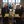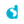0%
0%

# PLL Design using Delfino Launchpad

This project details the design of a Single Phase Phase Locked Loop

Similar projects worth following
3.5k views
In power applications, we often require a PLL to lock the grid frequency to be used for control applications. This article details the design of Single Phase PLL based on TI's Application Note: http://www.ti.com/lit/an/sprabt3/sprabt3.pdf

## CONTROL DESCRIPTION:

Consider the control diagram as shown above.

PLL generates two signals, One Sine and second Cosine. The cosine signal is feed back and multiplied with PLL input signal.

Let PLL input be

Let the cosine output of PLL be

The input to the Notch filter,

Where wi = Frequency of PLL input signal, wo = PLL set Frequency (at steady state) and K = Magnitude of PLL input signal

Using trigonometry, we get

If wi = 50Hz (Ideal grid freq. in India) & wo = 50Hz (at steady state), We have a twice frequency component (wi+wo) in the above equation. To remove this, the Notch filter is tuned at 100Hz. The input to PI thus becomes,

At steady state, wi = wo,

For small values of Theta, Sin(theta) = theta. Therefore,

Hence, the input to PI (loop filter) is approximately the Phase difference b/w PLL input and Output Signal.

## NOTCH FILTER DESIGN:

A Biquad Notch filter will suffice for our application.

The Direct Form II equation for a biquad filter is given as,

where x(n) = Present Input and y(n) = Present Output

The numerator and denominator coefficients can be computed using the excel sheet given below (in "files" section of this page).

## PI LOOP FILTER DESIGN:

One way to design a PI is using the so called Velocity Algorithm,

We only need to store present and previous sample values.

## SINE/COSINE GENERATION:

We can generate sine/cosine using integration as explained in .

The EQUATIONS for SINE/COSINE generation:

where Ts = Sampling Frequency, Wset = PLL set frequency

## PLL TESTING:

For testing the PLL, i created a Variable Frequency Sine wave generator using CLA (Control law accelerator) of the Delfino Microcontroller. This generator runs in backend and can be used generate Sine wave input for PLL testing.

Watch the PLL in action in the video below:

### PLL_NOTCH.m

Matlab Script for the Single Phase PLL

plain - 4.19 kB - 01/14/2016 at 08:19

### Notch Filter Coefficients (2p2z).xlsx

Find Coefficients for Notch Filter

sheet - 11.23 kB - 01/14/2016 at 08:15

• ### PLL Testing + Python Waveform Plotter

Jaspreet Singh02/23/2016 at 16:29 0 comments

A created a python based waveform plotter for viewing the PLL waveforms on my laptop. The source files are available on my github repository. Also, watch python waveform plotter in action in the video below.

Share

## Similar Projects

Project Owner Contributor

### Affordable Spectrum AnalzyerDan Kisling

Project Owner Contributor

### How to Create a Peak to Peak Frequency MonitorDialog Semiconductor

# Does this project spark your interest?

Become a member to follow this project and never miss any updates